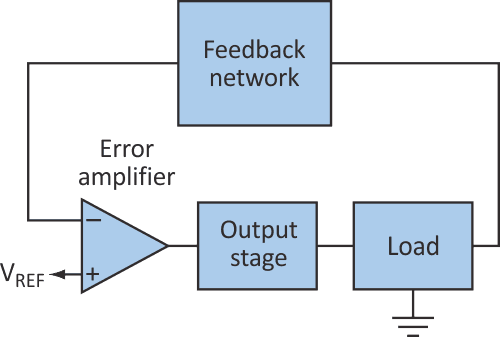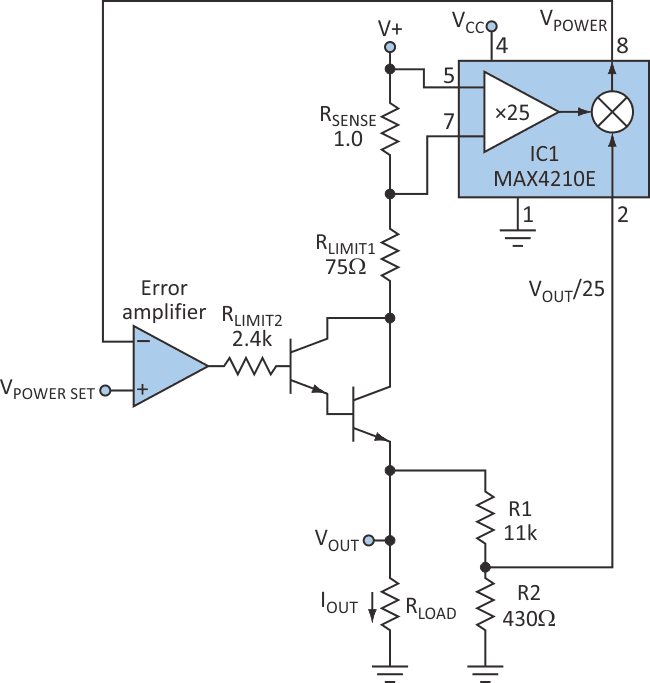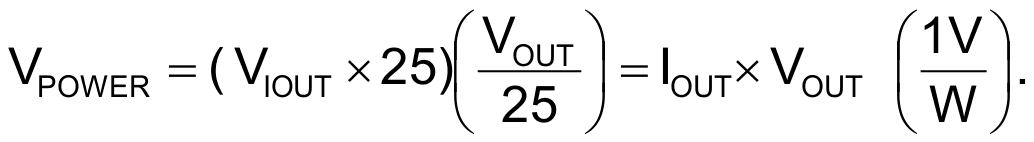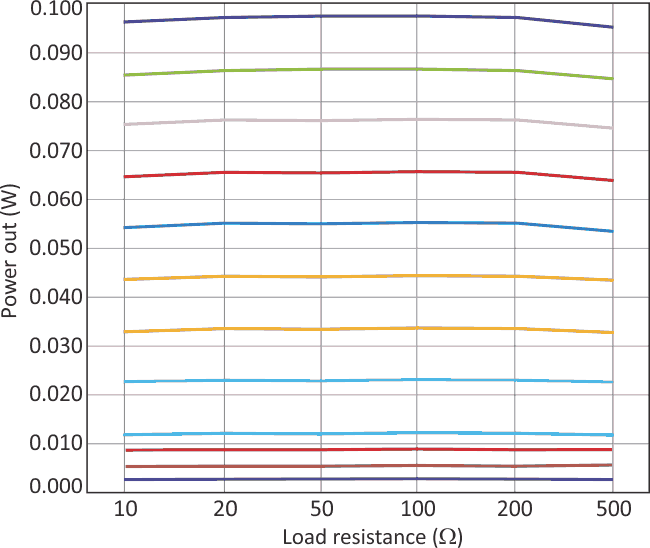# Supply Constant Power Level To A Varying Load Impedance

## Maxim MAX4210

How do you deliver constant power when the load impedance isn't constant? That problem arises, for example, in trying to maintain a warm LCD display on an outdoor gas pump in cold climates. As the heating element changes temperature, its resistance changes as well. That variation is characterized by the temperature coefficient of the heating element. If you apply a constant voltage, power delivered to the load will vary inversely with the load resistance.

In many applications, variations in applied power are acceptable within limits. To reduce variations, however, you must allow the applied voltage to vary with load resistance. The approach shown here uses a conventional control loop (Fig. 1).Figure 1. A typical feedback loop for a voltage regulator can be adapted to create a power regulator for a load with varying impedance.

For a linear voltage regulator, the error amplifier compares a sample of output voltage to a reference voltage and forces the output stage to deliver load current at a constant voltage. To maintain constant power instead of constant voltage, though, you must incorporate power into the feedback network. The error amplifier then compares a sample of output power against the reference and drives the output stage to keep them equal.

The power regulator in Figure 2 delivers a constant power that's linearly proportional to the voltage applied at VPOWER_SET. (The ratio is: power out/VPOWER_SET = 1 W/V.) The regulator delivers up to 100 mW, driving loads from 10 to 500 Ω with voltages up to 10 V and currents up to 100 mA.Figure 2. The feedback loop in the power regulator ensures that the power to the varying load remains relatively constant.

The key to this circuit is a high-side power and current monitor (IC1), which includes the circuitry needed to generate a feedback voltage proportional to the instantaneous load power. IC1 contains a current monitor that measures load current, a buffer that measures load voltage, and an analog multiplier that multiplies the two together, producing an output voltage proportional to load power. The current monitor is a high-side type, in which the sense resistor is connected to the hot side of the load instead of the low (ground) side. This avoids adding unwanted resistance in the ground path.

The monitor in this example (one of several available) has a gain of 25 in its current-sense amplifier. Therefore, for a 1-Ω sense resistor, the amplifier output is 25 V per ampere of current. The error amplifier drives a high-gain Darlington pair to minimize base current, which flows in the load but not through the sense resistor.

The second input for the power measurement is load voltage, which is monitored via a resistor divider (R1-R2) with a 1:25 ratio. That voltage is internally multiplied with the current signal to produce an output proportional to load power:By controlling drive to the output stage, the error amplifier forces the IC1 output (proportional to load power) to equal the reference signal at VPOWER_SET.

The V+ supply voltage (18 V) limits the maximum load voltage to approximately 15 V. RLIMIT1 and RLIMIT2 limit the load current to approximately 120 mA.

This circuit maintains a fairly constant load power over a 50:1 variation in load and for a number of different POWER_SETtings (Fig. 3).Figure 3. The power output for the circuit in Figure 2 remains well regulated for a wide range of loads and for various values of VPOWER_SET, which range from 0.0021 (bottom) to 0.0300 V (top).

Though this example circuit is designed for low-power applications (to 100 mW) across a wide range of load impedances, it can easily be scaled to handle wide ranges of load current, voltage, and power. To do that, adjust the current-sense resistor for a mid-range value of approximately 50 mV (voltage across this resistor must be less than 150 mV).

Similarly, the R1-R2 ratio should be adjusted so that the voltage presented to IC1 is roughly 500 mV (200 mV to 1 V yields the specified accuracy for IC1). Other versions of IC1 incorporate different values of gain, which affects the ratio of power output to the applied VPOWER_SET level.

Finally, you must consider and modify as required the power-handling capability of RLIMIT1 and the output transistor. This technique isn't limited to the linear regulator shown. It applies equally well to many switching regulators, which allow for considerably higher output power and higher efficiency.

## Materials on the topic

electronicdesign.com